### 自行车发电机

MuggleV 发布于 2015-06-26 16:48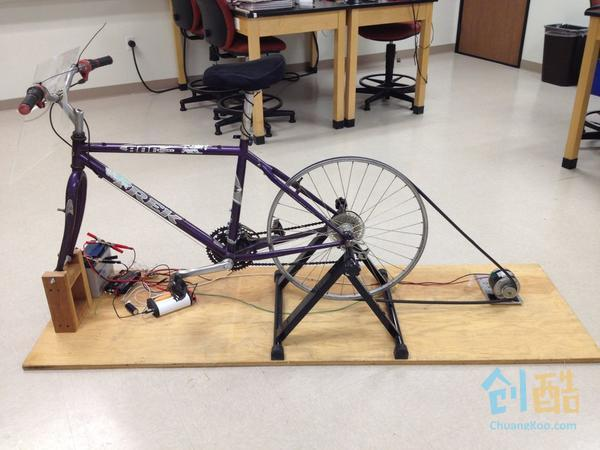#### Step 1： 固定自行车架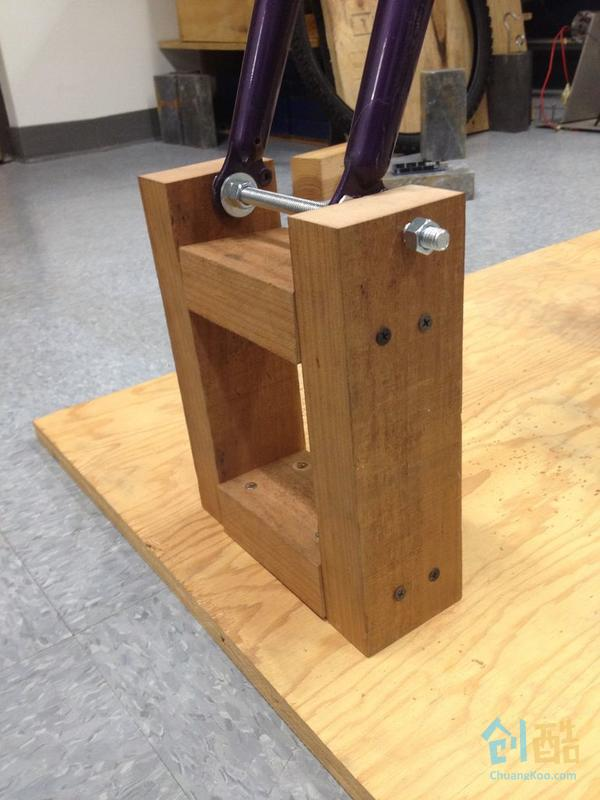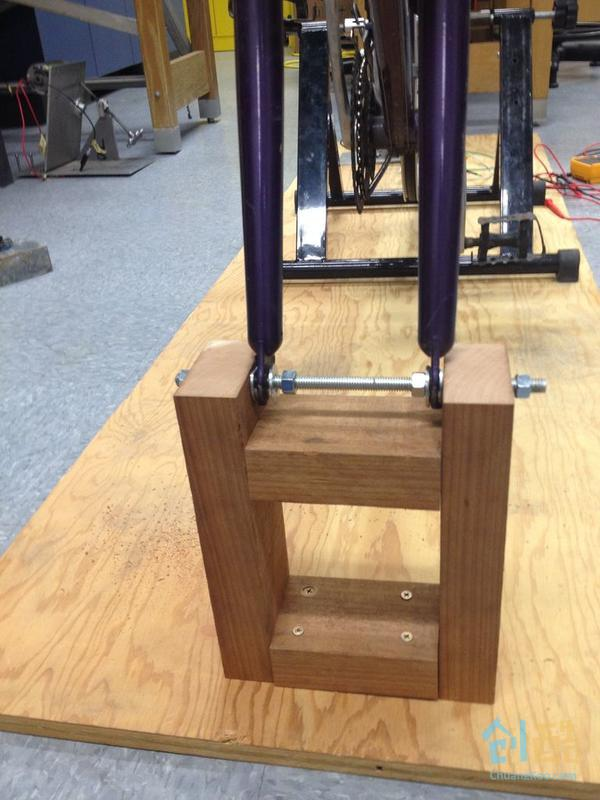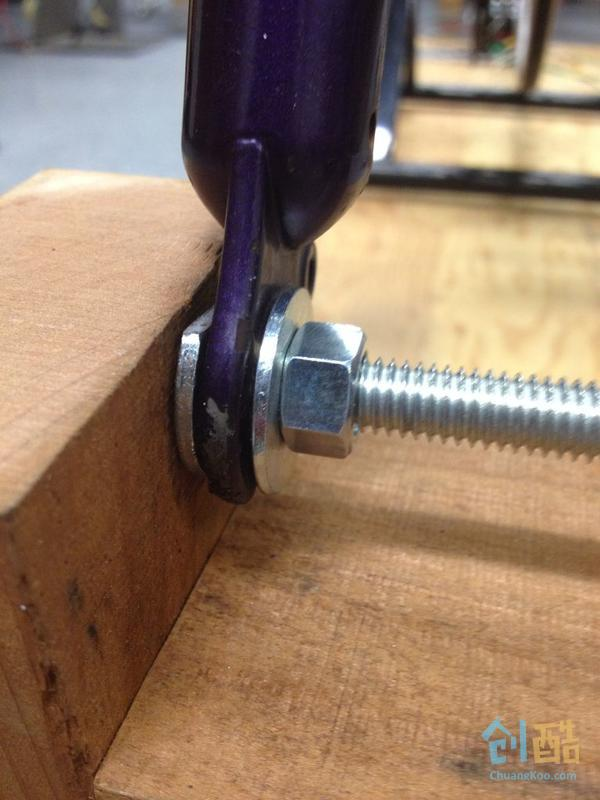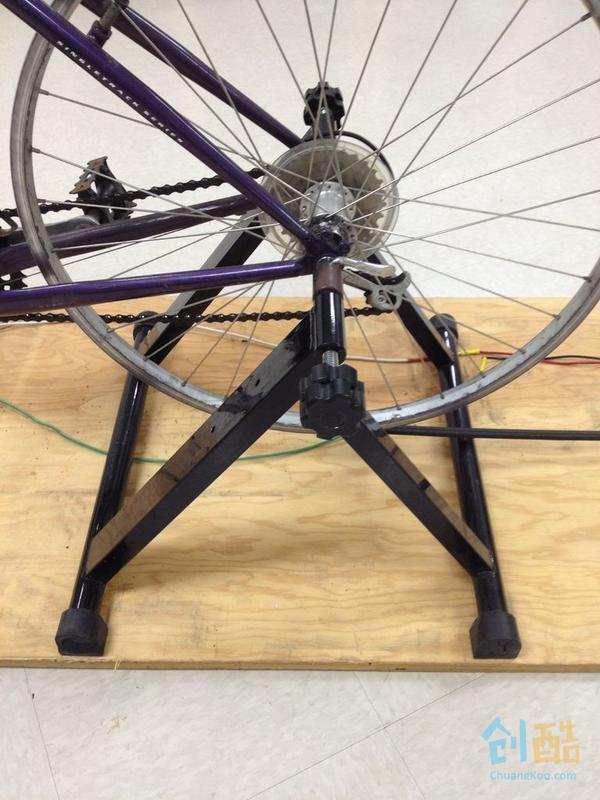#### Step 2： 连接皮带轮和电机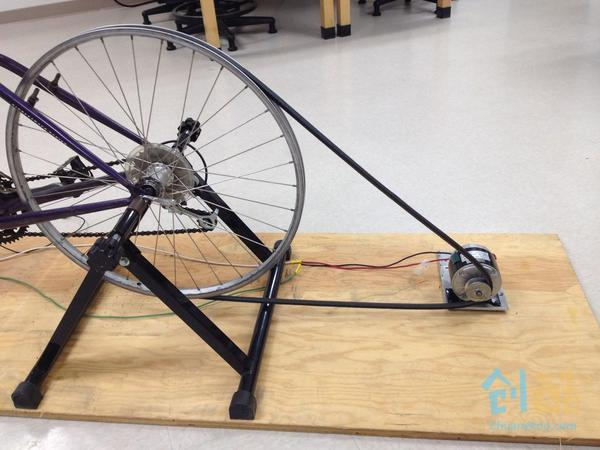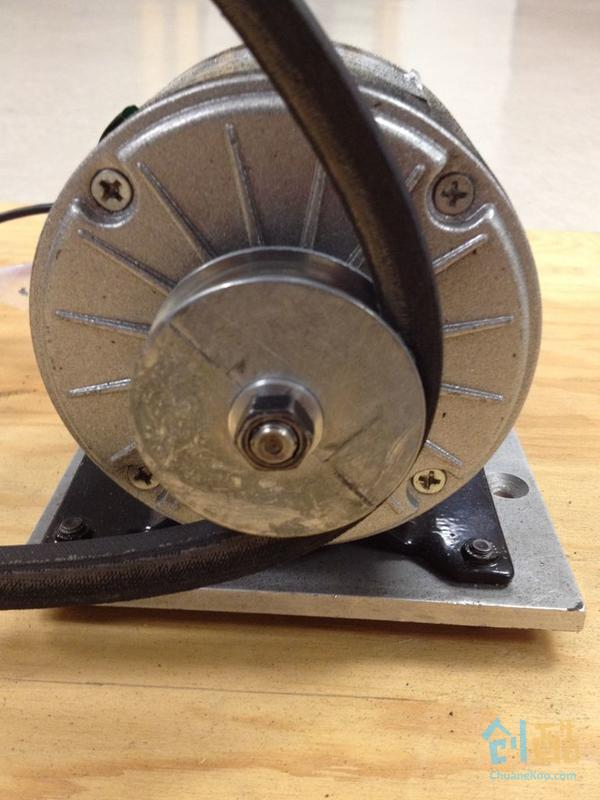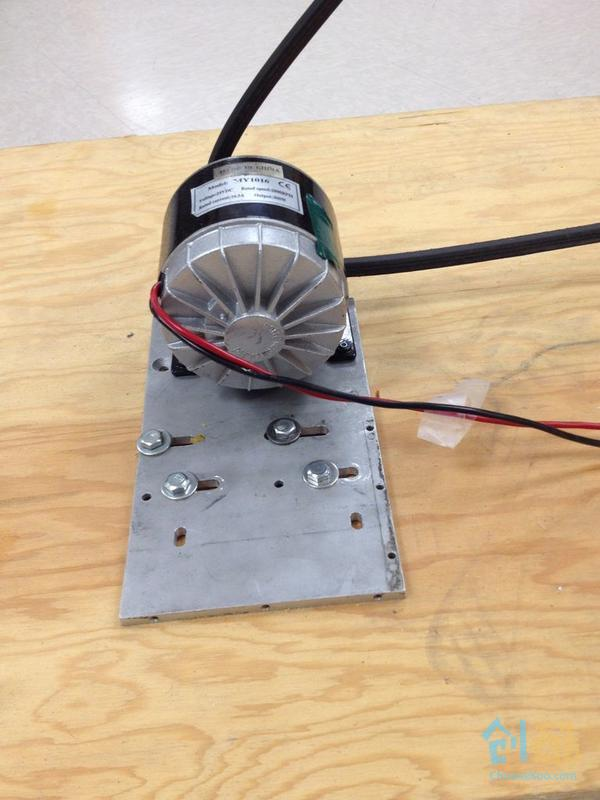#### Step 4： 连接电机和充电器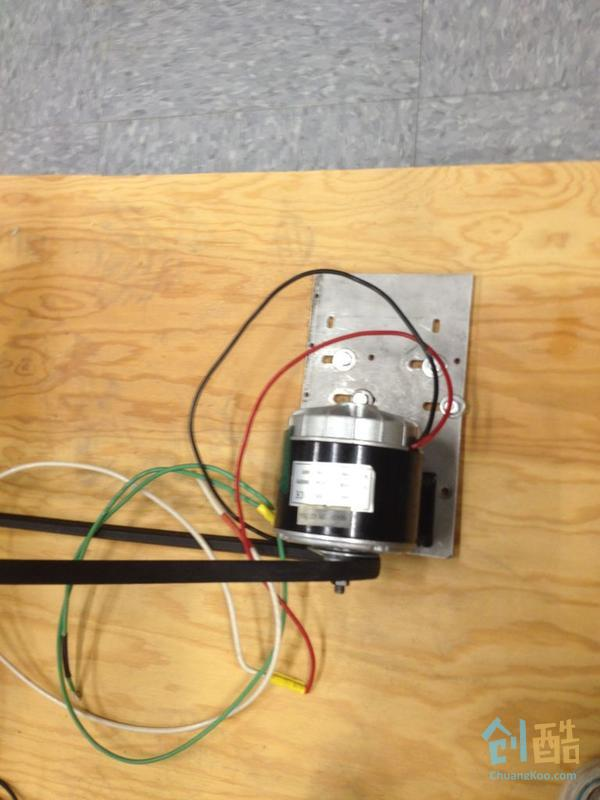#### Step 5： 连接充电器和蓄电池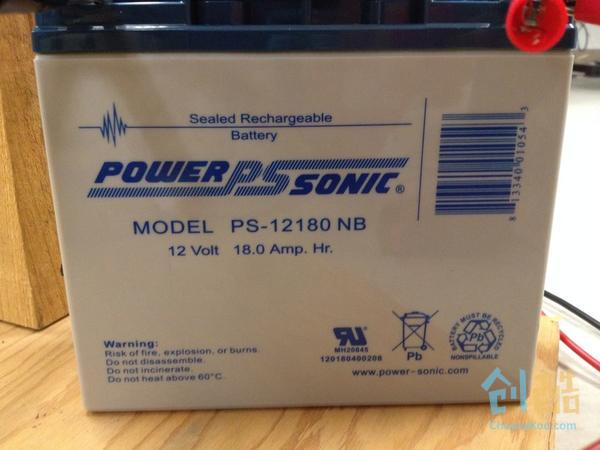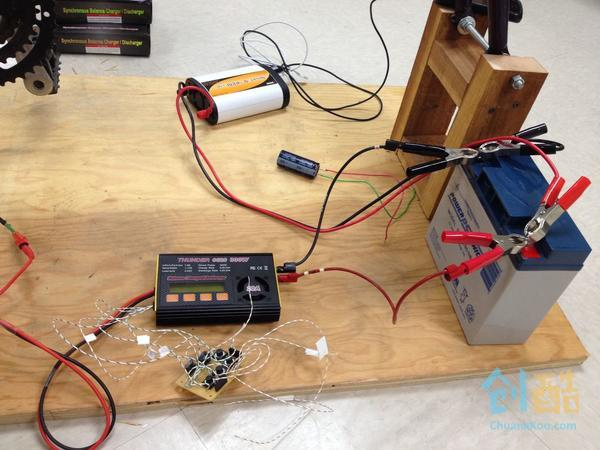http://www.power-sonic.com/images/powersonic/sla_batteries/ps_psg_series/12volt/PS-12180_12_Sept_10.pdf

#### Step 6： 连接电池和逆变器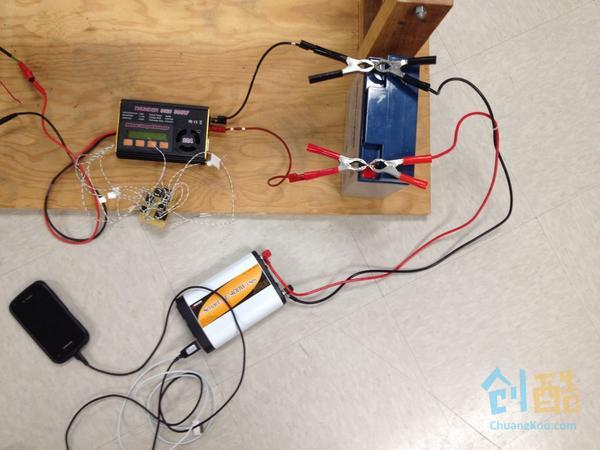#### Step 8： 改装充电控制器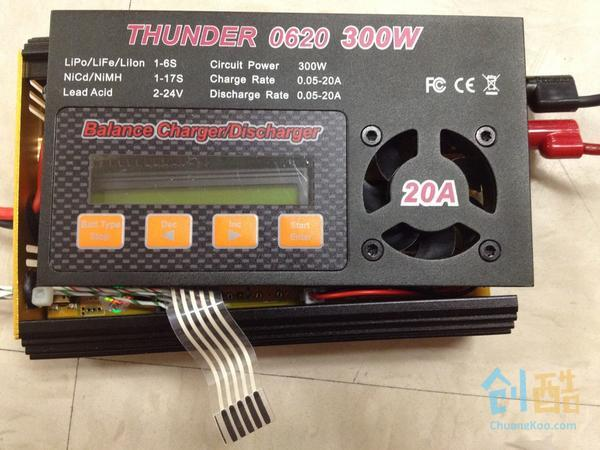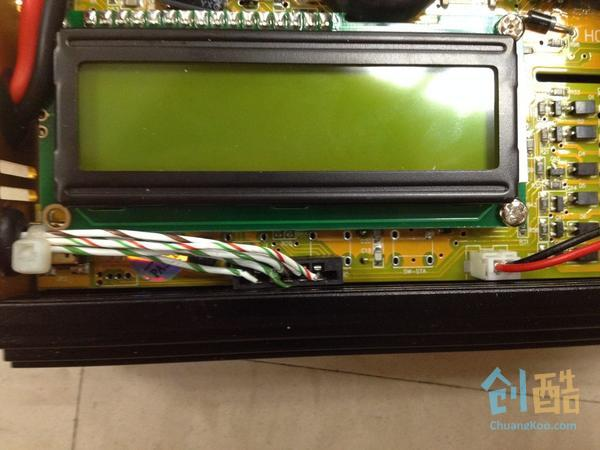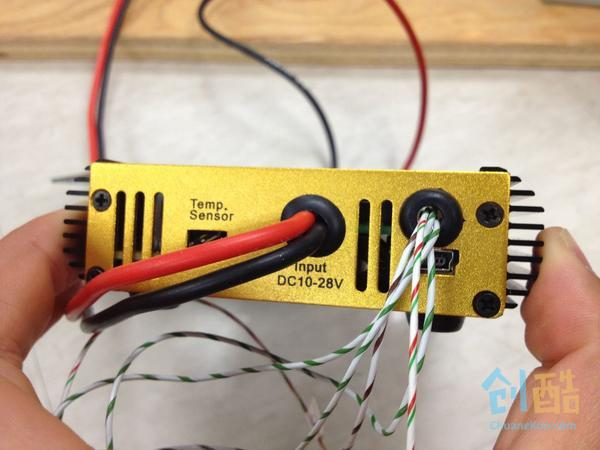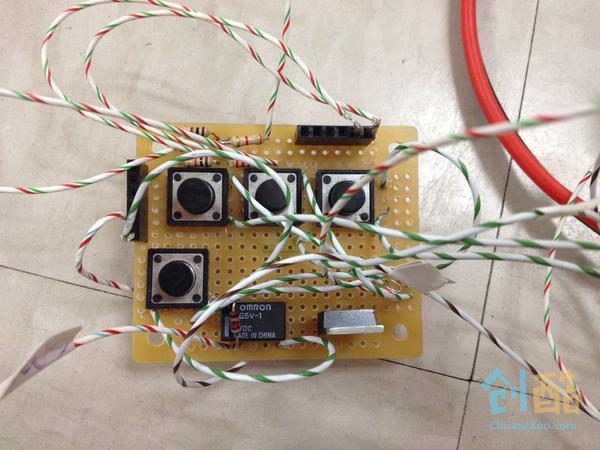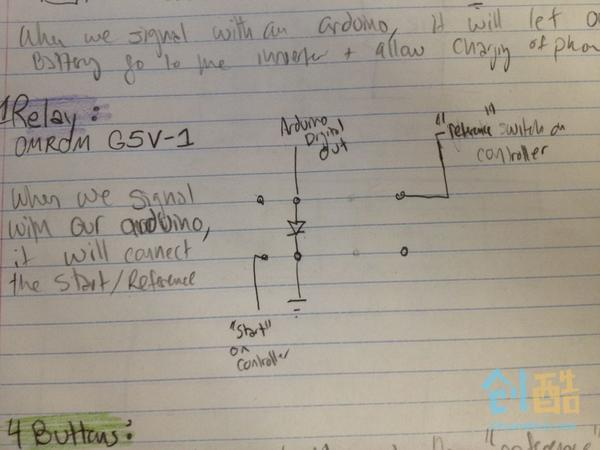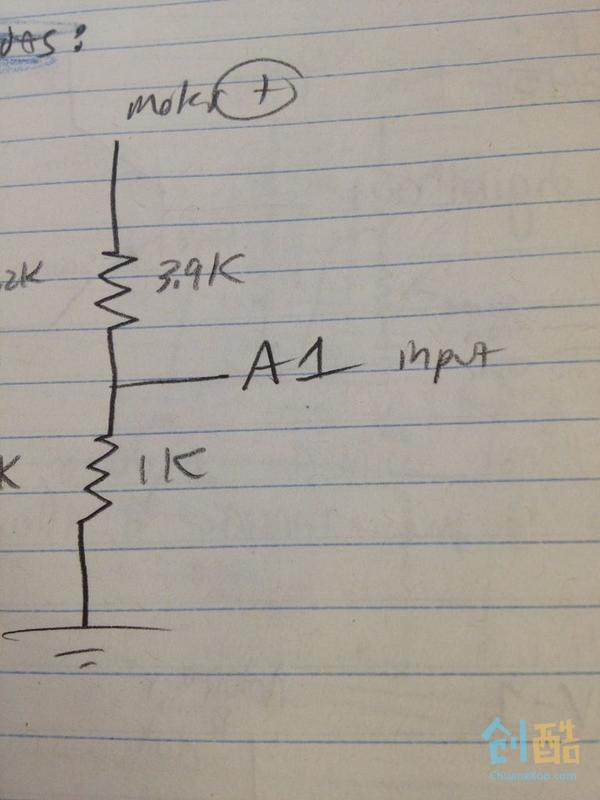```void loop(){
while(!started){

startCharger();

}

void startCharger(){

if (motorVoltage>12 && hasBeenOnForLong){

digitalWrite(relayOut, HIGH); //which really is equivalent to pressing Start
started=true;

}

else if (motorVoltage>12){

//say it has been on for longer

}

else{

//reset hasBeenOnForLong to the beginning

}

}```

#### Step 9： 显示电池电量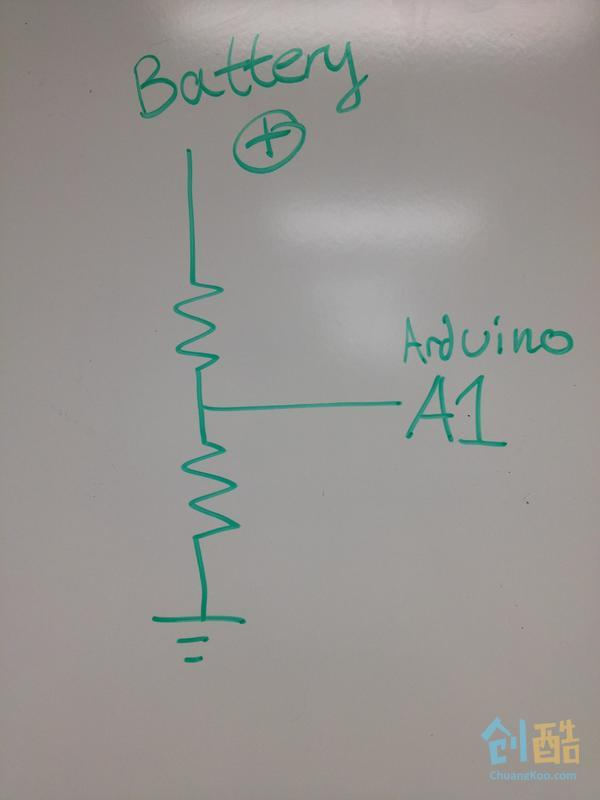#### Step 10： 保存电池电量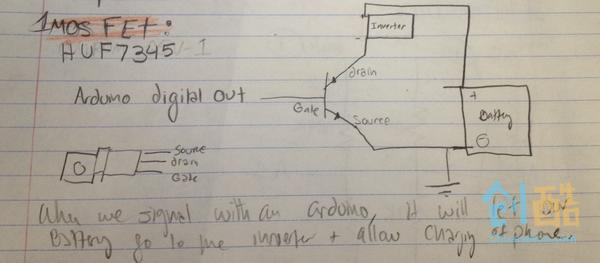```String allowBatteryAccess(){
if(currentMillis-startMillis>(1000*120)){ //if 2 minutes of time has elapsed

digitalWrite(allowBattery, HIGH);

return "Your device has started to charge. Keep Peddling";

}

return " "

}```

#### Step 11： 电路板布局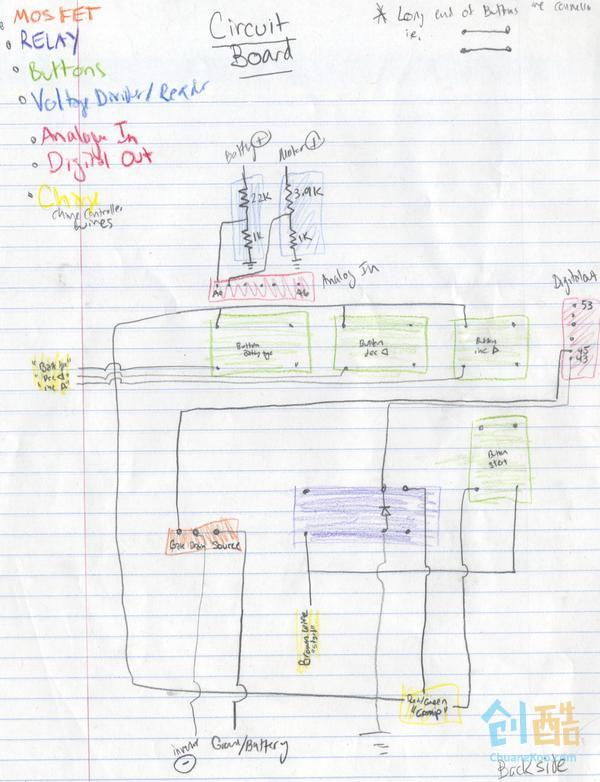```/* Sallie and Sasha charger programming

*/

// include the library code:
#include <Wire.h>

//PinValues we need to change these
int motorVoltagePin= A0; // voltage output from motor just for fun
int batteryVoltagePin= A1; //voltage of battery that give percent charge
int relayOut=23; //for automatically pressing start button on charger
int allowBattery=24; //for allowing current through the charger

//variables
unsigned long startMillis = 0;
unsigned long currentMillis = 0;
int on = 0;
boolean started = false;
float motorVoltageValue=0;
float batteryPercent=0;
float batteryVoltage=0;

//constants
const float referenceVolts = 5;
const float R1 = 3900; // value for a maximum voltage of 30 volts
const float R2 = 1000;
const float RB1 = 2200; // value for a maximum voltage of 15 volts
const float RB2 = 1000;
const float resistorFactorA = 1023.0 / (R2/(R1 + R2));
const float resistorFactorB = 1023.0 / (RB2/(RB1 + RB2));

void setup(){
Serial.begin(9600);
}

void loop(){
while(!started){
startCharger();
startMillis = millis();
}
Serial.println("You've Started the Charging");
Serial.println("The voltage you are generating is this:");

Serial.println("The battery is charged this many %");
Serial.println(batteryVoltagePercent());
Serial.println(allowBatteryAccess());
}

String allowBatteryAccess(){
if(currentMillis-startMillis>(1000*120)){  //if 2 minutes of time has elapsed
digitalWrite(allowBattery, HIGH);
return "Your device has started to charge. Keep Peddling";
}
return "";
}

void startCharger(){
Serial.println("Keep pedaling to start charging");
motorVoltage();
if((motorVoltageValue>9) && (on>100)){ //if user has been pedaling at a good speed switch relay on
digitalWrite(relayOut, HIGH);        //which really is equivalent to pressing Start
started=true;                        //on the front panel of the charger
delay(1000);                         //Hold button for a minute
}
if(motorVoltageValue<2){ //reset the on value if you stop biking, restart the counter
on=0;
}
if(motorVoltageValue>9){ //if you are actually biking at a good speed increase the on count
on++;
}
digitalWrite(relayOut, LOW);
}

float volts = (val / resistorFactorB) * referenceVolts ; // calculate the ratio
batteryVoltage =volts;  // print the value in volts
return batteryVoltage;
}

float batteryVoltagePercent(){
if(batteryVoltage<12){
batteryPercent=.1;
}
if(batteryVoltage<12.5){
batteryPercent=.2;
}
if(batteryVoltage<13){
batteryPercent=.25;
}
if(batteryVoltage<13.5){
batteryPercent=.6;
}
if(batteryVoltage<14){
batteryPercent=.8;
}
if(batteryVoltage<15){
batteryPercent=1;
}
}

//say we have a max of 24 volts coming from the motor, we want to scale that down by a factor of 5
//to make sure our arduino can read it (it can read 0 to 5 volts) thus we make a voltage divider where R1
//is four times as large as R2 so the voltage is 1/5 of the read out of the motor.  We want a small load
//so we make our resistors very large
float motorVoltage(){
float volts = (val / resistorFactorA) * referenceVolts ; // calculate the ratio
motorVoltageValue =volts;  // print the value in volts
return motorVoltageValue;
}```

#### Step 12： 进度&后续打算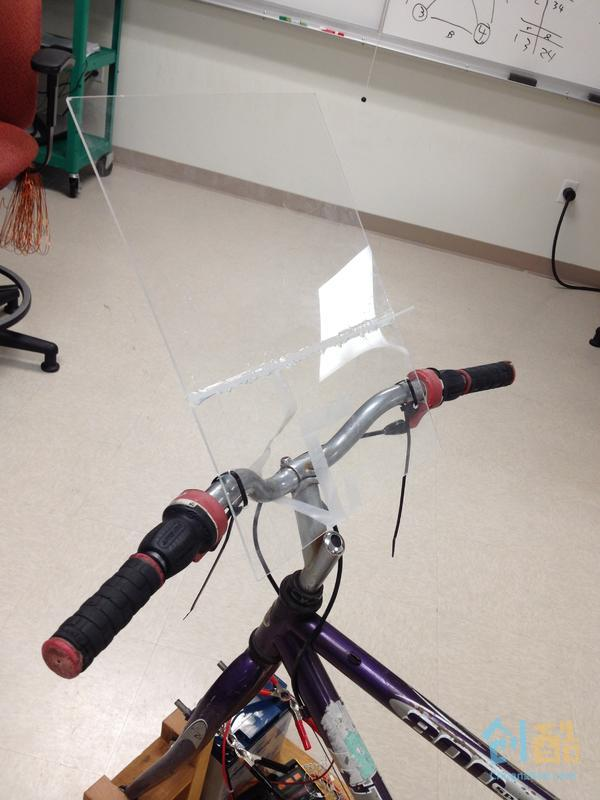fxyc872015-08-01 18:16:19

2粉丝 1作品 0随笔

## 作者的最新作品